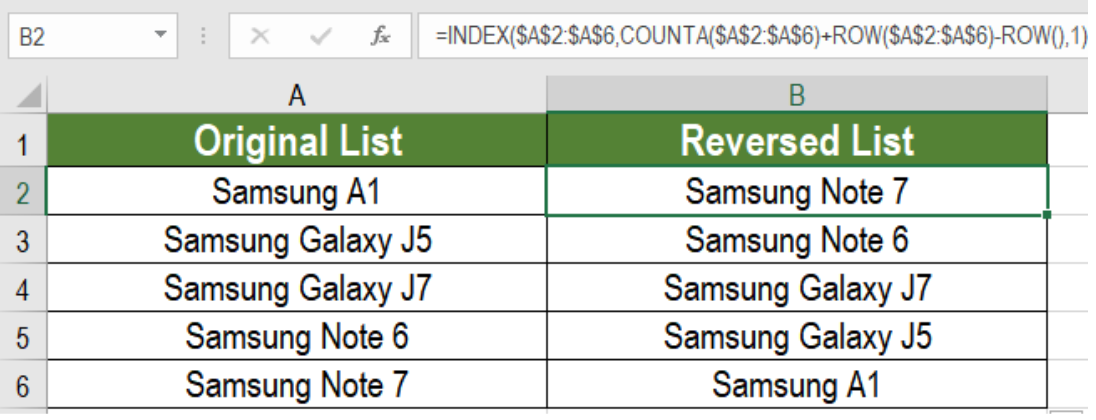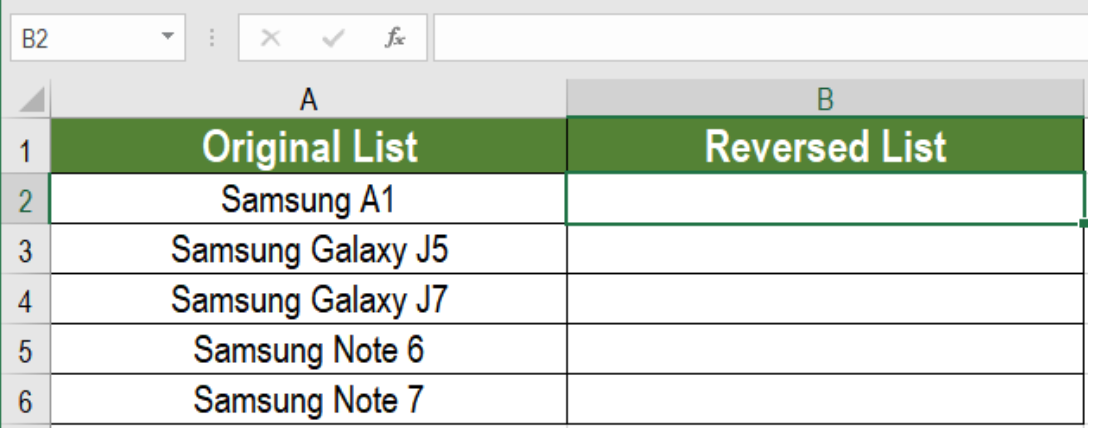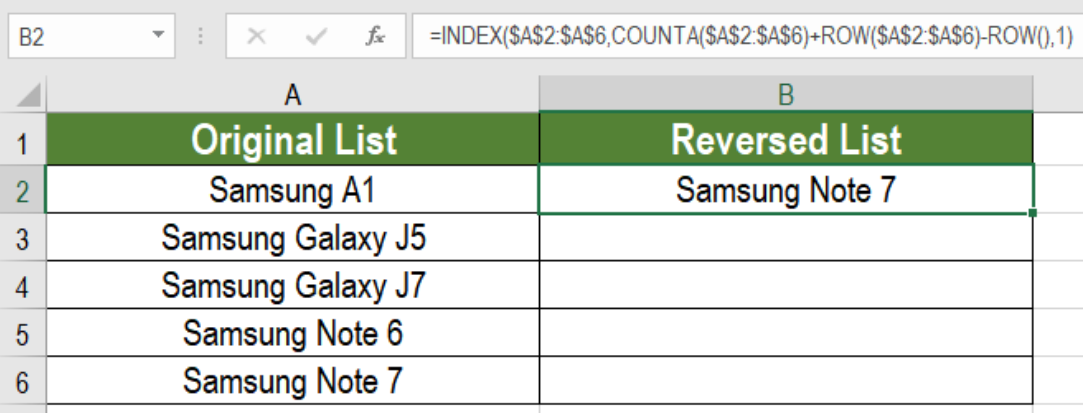Get instant live expert help with Excel or Google Sheets“My Excelchat expert helped me in less than 20 minutes, saving me what would have been 5 hours of work!”

#### Post your problem and you’ll get expert help in seconds.

Your message must be at least 40 characters
Our professional experts are available now. Your privacy is guaranteed.

# Reverse a list or range

After completing a list, it is hard to manually reverse all the data. It takes a lot of time and effort. But in excel, we can do it using three commonly used functions. We can use the  INDEX, COUNTA and ROW functions together to reverse a list or string. In this tutorial, we will learn how to reverse a list or string in Excel.Figure 1: Example of How to Reverse a List or String in Excel

## Generic Formula

`=INDEX(List,COUNTA(List)+ROW(List) - ROW(),1)`

## How the Formula Works

Here, the COUNTA function takes the list as an argument and returns the number of non blank items. We add this number  to the first ROW function. It also takes the list as an argument and returns the first row number of the list. The second ROW function returns the current row number where the formula is working.

We subtract this value from the summation of the previous values. All this goes into the second argument of an INDEX function. The first argument is the list itself and the row number is set to 1. The ROW function provides a number that is increasing.

We use this to create a row number that represents the last item of the list and then gets toward the first item. Thus, the last item in the list gets a row number by the first instance. The second to last item gets a row number by the second instance, and so on. This reverses the entire list.

## Example

The following example contains a list of Samsung phones in column A.Figure 2: The List to be Reversed

To reverse it in column B we need to:

• Go to cell B2. Click on it with your mouse to select it.
• Then, we have to assign the formula `=INDEX(A2:A7,COUNTA(A2:A7)+ROW(A2:A7)-ROW(),1)` to cell B2.
• Lastly, we need to drag the fill handle from cell B2 to B6 to copy the formula to the cells below.Figure 3: Example of Applying the Formula to the List

Now, the last entry in the list “Samsung Note 7” will now appear in the beginning of the list. This will in turn reverse the entire list.

Most of the time, the problem you will need to solve will be more complex than a simple application of a formula or function. If you want to save hours of research and frustration, try our live Excelchat service! Our Excel Experts are available 24/7 to answer any Excel question you may have. We guarantee a connection within 30 seconds and a customized solution within 20 minutes.

### Did this post not answer your question? Get a solution from connecting with the expert.Another blog reader asked this question today on Excelchat:
Related blogs
Solution examplesI need a formula to calculate the MAX of a range of 167 rows in column D, then find the MAX of the next 167 rows in column D and so on continuously through the entire sheet. Example MAX D2:D169, D170:D337, D338:D505 etc...
Solved by I. Q. in 60 minsHow can I automatically change different cells and the information they display if we select different option from a drop down list?
Solved by V. U. in 59 minsI need to create a rows in Sheet 1 where Sheet 2 column c has values in Sheet 2 column D that are greater than .01 and leave out items in Sheet 1 where Sheet 3 column D are = 0 (without using a filter, then copy/paste).
Solved by I. Y. in 21 minsI need help on index and match where the return is not correct
Solved by B. B. in 20 minsI have a database extract that is showing the same clients multiple times in the list. How can I write a formula in excel to look at the column with the client names and return only unique records on another sheet? I want to run this over several files so wanting to avoid using pivot tables which is how I would normal do this.
Solved by X. J. in 20 mins## Subscribe to Excelchat.coAnother blog reader asked this question today on Excelchat: